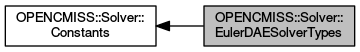OpenCMISS-Iron FORTRAN API Documentation
OPENCMISS::Solver::EulerDAESolverTypes

The Euler solver types for a differential-algebriac equation solver. More...

Collaboration diagram for OPENCMISS::Solver::EulerDAESolverTypes:Variables

integer(intg), parameter, public opencmiss_iron::cmfe_solver_dae_euler_forward = SOLVER_DAE_EULER_FORWARD
Forward Euler differential equation solver. More...

integer(intg), parameter, public opencmiss_iron::cmfe_solver_dae_euler_backward = SOLVER_DAE_EULER_BACKWARD
Backward Euler differential equation solver. More...

integer(intg), parameter, public opencmiss_iron::cmfe_solver_dae_euler_improved = SOLVER_DAE_EULER_IMPROVED
Improved Euler differential equation solver. More...

Detailed Description

The Euler solver types for a differential-algebriac equation solver.

OPENCMISS::Solver::Constants,OPENCMISS

Variable Documentation

 integer(intg), parameter, public opencmiss_iron::cmfe_solver_dae_euler_backward = SOLVER_DAE_EULER_BACKWARD

Backward Euler differential equation solver.

OPENCMISS::Solver::EulerDAESolverTypes,OPENCMISS

Definition at line 6228 of file opencmiss_iron.f90.

 integer(intg), parameter, public opencmiss_iron::cmfe_solver_dae_euler_forward = SOLVER_DAE_EULER_FORWARD

Forward Euler differential equation solver.